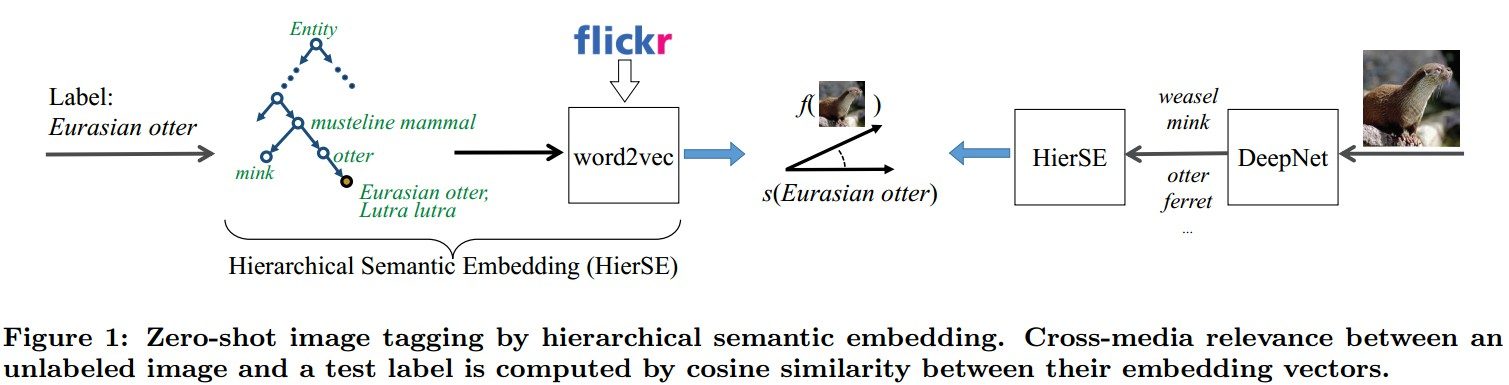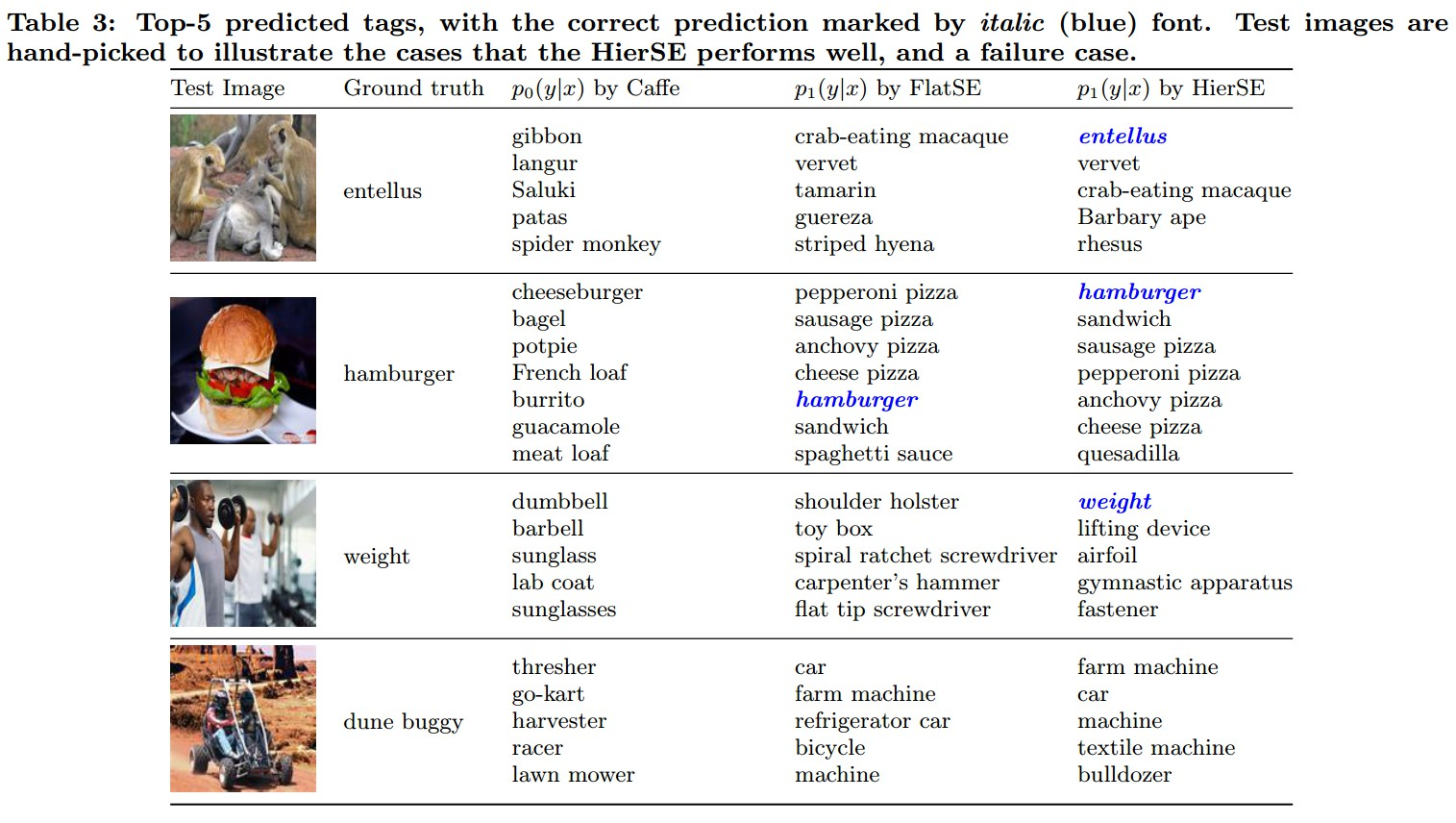AIHGF23
2018/05

# 论文阅读 - Zero-shot Image Tagging by Hierarchical Semantic Embedding

zero-shot learning 不是寻找图像和目标标签的直接映射关系，其关键在于在图像和标签之间引入中间层，使得新标签也可在这层进行表示，即使没有该标签的图像样本.

## 2. 语义嵌入模型

${ f(x) := \frac{1}{Z} \sum_{t=1}^{T} p_{0}(y(x,t)|x) \cdot s(y(x,t)) }$

${ p_{1}(y|x) := cos(f(x), s(y)) }$

## 3. 层次语义嵌入

${ s_{hi}(y) = \frac{1}{Z_{hi}} \sum_{y^\prime \in {y} \bigcup super(y)}w(y^\prime|y) \cdot s(y^\prime) }$## 4. Results## 参考文献

 - Zero-shot learning by convex combination of semantic embedding. In ICLR, 2014

Last modification：October 10th, 2018 at 01:16 am

#### Leave a Comment